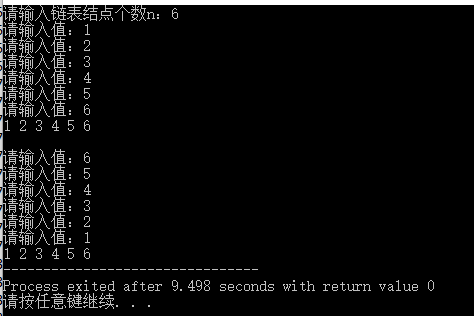• 编写一程序实现将用户输入的一串字符以反响形式输出。比如，输入的字符串是：abcdefg，输出为：gfedcba。
• # include # include # include typedef struct node { int data ; struct node * next ;...//尾插法，实现反向输出链表 return 0 ; } 运行结果：
#include <stdio.h>
#include <stdlib.h>
#include <malloc.h>

typedef struct node{
int data;
struct node *next;
}Node, *pNode;

pNode rear_create(int n){//尾插法建立
pNode head = (pNode)malloc(sizeof(Node));
pNode rear = head;//注意与头插法不同地方
if(head == NULL)
{
printf("存储分配失败！\n");
exit(-1);
}
else{
for(int i=0; i<n; i++){
pNode pNew = (pNode)malloc(sizeof(Node));
if(head == NULL)
{
printf("存储分配失败！\n");
exit(-1);
}
else{
printf("请输入值：");
scanf("%d", &(pNew->data));
rear->next = pNew;//注意与头插法不同地方
pNew->next = NULL;//注意与头插法不同地方
rear = pNew; //注意与头插法不同地方
}
}
}
return head;
}

pNode head_create(int n){//头插法建立
pNode head = (pNode)malloc(sizeof(Node));
head->next = NULL;//注意与尾插法不同地方
if(head == NULL)
{
printf("存储分配失败！\n");
exit(-1);
}
else{
for(int i=0; i<n; i++){
pNode pNew = (pNode)malloc(sizeof(Node));
if(head == NULL)
{
printf("存储分配失败！\n");
exit(-1);
}
else{
printf("请输入值：");
scanf("%d", &(pNew->data));
pNew->next = head->next;//注意与尾插法不同地方
head->next = pNew;//注意与尾插法不同地方
}
}
}
return head;
}

void traverse_list(pNode head){
pNode p = head->next;
while(p != NULL){
printf("%d ", p->data);
p = p->next;
}
}

int main() {
int n;
printf("请输入链表结点个数n：");
scanf("%d", &n);

pNode h1 = rear_create(n);
traverse_list(h1);	//尾插法，实现顺序输出链表
printf("\n\n");
pNode h2 = head_create(n);
traverse_list(h2);	//尾插法，实现反向输出链表

return 0;
}

运行结果：展开全文算法
• 无法正确反向输出链表 <code>#include <stdio.h> #include "string.h" #include "stdlib.h" typedef struct node { int data; struct node* next; }Node; int main() { struct node *...有问必答
• C语言 将字符串反向输出 #include <stdio.h> #include <stdlib.h> #include <string.h> //字符串反向输出 void reverse(char *arr) { int temp, i; int len; len = strlen(arr); //获得...
C语言 将字符串反向输出
#include <stdio.h>
#include <stdlib.h>
#include <string.h>
//字符串反向输出

void reverse(char *arr)
{
int temp, i;
int len;

len = strlen(arr); //获得字符串的长度
for( i = 0; i < len / 2; i++)
{
temp = arr[i];
arr[i] = arr[len - i - 1];
arr[len - i - 1] = temp;
}

}

int main(void)
{
char s = "abcdefg";
int i;

reverse(s);
//		输出
puts(s);
system("pause");
return 0;
}



展开全文字符串
• 问题描述：请使用C语言编程实现反向输出一个链表。 问题分析： 线性表的链式存储表示的特点是用一组任意的存储单元存储线性表的数据元素（这组存储单元可以是连续的，也可以是不连续的）。因此，为了表示每个数据...
问题描述：请使用C语言编程实现反向输出一个链表。

问题分析：

线性表的链式存储表示的特点是用一组任意的存储单元存储线性表的数据元素（这组存储单元可以是连续的，也可以是不连续的）。因此，为了表示每个数据元素与其直接后继数据元素 之间的逻辑关系，对数据元素 来说，除了存储其本身的信息之外，还需存储一个指示其直接后继的信息（即直接后继的存储位置）。由这两部分信息组成一个"结点"（如概述旁的图所示），表示线性表中一个数据元素。线性表的链式存储表示，有一个缺点就是要找一个数，必须要从头开始找起，十分麻烦。循环链表是与单链表一样，是一种链式的存储结构，所不同的是，循环链表的最后一个结点的指针是指向该循环链表的第一个结点或者表头结点，从而构成一个环形的链。

程序源码：

/*reverse output a list*/
#include "stdlib.h"
#include "stdio.h"
struct list
{ int data;
struct list *next;
};
typedef struct list node;
typedef node *link;
void main()
{ link ptr,head,tail;
int num,i;
tail=(link)malloc(sizeof(node));
tail->next=NULL;
ptr=tail;
printf("\nplease input 5 data==>\n");
for(i=0;i<=4;i++)
{
scanf("%d",&num);
ptr->data=num;
head=(link)malloc(sizeof(node));
head->next=ptr;
ptr=head;
}
ptr=ptr->next;
while(ptr!=NULL)
{ printf("The value is ==>%d\n",ptr->data);
ptr=ptr->next;
}}


展开全文• ## C语言：反向输出

千次阅读 2011-04-18 23:10:00
Code: #include void main() { void swap(int x[],int n); int a = {1,2,3,4,5,6,7,8,9,0}; int i; for (i=0;i {


Code:

#include <stdio.h>

void main()
{
void swap(int x[],int n);
int a = {1,2,3,4,5,6,7,8,9,0};
int i;
for (i=0;i<10;i++)
{
printf("%d   ",a[i]);
}
swap(a,10);
for(i=0;i<10;i++)
{
printf("%d,",a[i]);
}
}
void swap(int x[],int n)
{
int j,tmp,p;
int m;
m = (n-1)/2;
for(j=0;j<=m;j++)
{
p = n-j-1;
tmp = x[j];
x[j] = x[p];
x[p] = tmp;
}
}

展开全文语言 c
• #include <stdio.h> void Input() ... printf("反向输出："); putchar(ch); } int main(void) { printf("请输入一个带感叹号字符串："); Input(); printf("\n"); return 0; } ...递归法
• 反向输出数值 给一个正整数， 要求： 一、求它是几位数， 二、逆序打印出各位数字。 示例中数组num2存储10位，所以支持输入1~10位的正整数； //给一个正整数， //要求：一、求它是几位数，/ //二、逆序...
• c语言写的字符串的反向输出 没什么用 得分就行
• 在此使用一种比较通用的方法，先获取输入到数组(链表)，然后将数组(链表)反向再打印 #include #define MAX_CHAR_NUM 10 void main(){ int c,i,j,temp; char str[MAX_CHAR_NUM]; c = i = j = temp = 0; ...
• /反向输出给定的整数/ #include<stdio.h> void main() { int n; printf(“please input the number:\n”); void int_turn(int); scanf("%d",&n); int_turn(n); } void int_turn(int n) { int...编程语言
• C语言代码实现 #include <stdio.h> #include <stdlib.h> typedef struct DoubleCirNode{ int data; struct DoubleCirNode *next; struct DoubleCirNode *prior; }DoubleCirNode,*DCList链表 单链表 算法 指针
• 问题:请从键盘中读入三个字符,将他们反向输出! 代码1:(用scanf跟printf实现) #include <stdio.h> int main() { char ch1 , ch2 ,ch3; printf("please input three characters: "); scanf("%c %c %c",&ch1 ...
• 递归反向输出字符串 利用递归函数调用方式， 将所输入的5个字符，以相反顺序打印出来。 //利用递归函数调用方式， //将所输入的5个字符，以相反顺序打印出来。 #include <stdio.h> int main() { printf...
• 将一个四位数反向输出 #define _CRT_SECURE_NO_WARNINGS 1 int main() { int a, b; scanf("%d", &a); b = a % 10; b = (a % 100) / 10; b = (a % 1000) / 100; b = a / 1000; for (int i ...
• #include<stdio.h> #include<stdlib.h> struct list { int data; struct list *next; }; int main() { struct list *head, *p, *tail;... tail = (struct list*)malloc(sizeof(struct ...
• 字符反向输出，今天所知道的知识有（%s是字符串，%c是字符，char是定义一个字符串）在for语句循环的时候，我没有很好的掌握下标的含义，所以导致在编写时遇到一些问题，另外再输出字符的时候要用（%c，n【m】）的...
• 目录1 题目2 分析2.1 方法1-原地逆置2.2 方法2-递归3 实现...反向输出一个链表，链表结构为： typedef int ElementType; typedef struct node { ElementType data; struct node *Next; } *List; 2 分析 2.1 方法...链表 算法 数据结构
• 编写一个递归函数，实现将输入的任意长度的字符串反向输出的功能。 例如输入字符串：ABCD，输出字符串：DCBA。【题目分析】 应用递归的思想有时可以很轻松地解决一些看似不太容易实现的功能。 本题就是利用...函数 递归 应用 string
• 一个递归反向输出链表 <code>#include <stdio.h> #include "string.h" #include "stdlib.h" typedef struct node { int data; struct node* next; }Node; int main() { struct node *...有问必答
• 反向输出一个链表-递归 程序分析 只需要反向输出即可，所以我们可以使用栈的特点，进行递归调用 递归过程如下： 主要代码 main.c typedef struct Node{ int data; struct Node *next; }Node,*LinkList; //带头...链表
• ![图片说明](https://img-ask.csdn.net/upload/202004/17/1587081750_280196.png) 请问哪里出错了，要怎么改？谢谢！ 输出了： The original string is Embedded Linux， The string afterward is
• C语言利用递归调用使字符串的反向输出，#为结束标志不做输出 #include<stdio.h> void print(){ char c; scanf("%c",&c); if(c!='#') print(); if(c!='#') printf("%c",c); } int main(){ print...
• 01 今天给大家安利一份代码，是关于字符串单词输入反向输出的实现效果的，就比如等你输入“welcome to China”，程序会经过处理输出“China to welcome”，具体怎么实现？这里小编用C语言和二维数组进行实现，具体......

# 反向输出c语言c语言 订阅